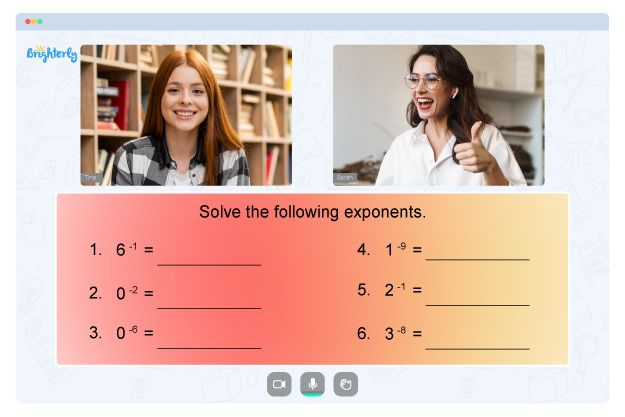# Ratios And Proportions Worksheets

Ratios are essential for kids when it comes to learning mathematics. They help introduce the idea of proportions to children, which they will later use when learning about algebra. Tools like a ratio and proportion worksheet allow them to understand measurements effectively.

Knowledge of ratio and proportion is a vital foundation for studying other mathematical concepts, and by practicing with ratio and proportion worksheets, kids can master these topics. A ratio compares two quantities of a similar kind, represented as a:b or a/b, and when two or more ratios are equal, they are in proportion. Ratios and proportions worksheets PDF with answers help teach kids to apply these concepts in everyday lives, such as when dealing with distances, weights, time, and heights.

## Ratio

A ratio comparing two similar quantities shows the number of portions in others. They are divided into two types: the part ratio and the whole ratio.

## Proportion

Proportion is comparing two quantities, also known as portion or amount, to the total. If the two ratios are equal, they are said to be proportional. There are two types of proportion: direct proportion and inverse proportion.

Math for Kids

Is Your Child Struggling With Math?
1:1 Online Math Tutoring## The Ratio and Proportion Worksheet

Ratios proportions worksheets are great for learning commonly recognized percentages, fractions, and decimal equivalents. Downloaded ratios and proportions worksheets in PDF format will produce a table of equivalent quantities rates for a student to work on. Kids may have to leave blank spaces or fill out the table. You can select different types of problems and have them in numerical or word format.

## Benefits of Ratio and Proportion Worksheets

Students develop practical knowledge through solving ratio and proportion worksheets as the concepts play an essential role in everyday life. Real-world examples include buying groceries, identifying price per kilogram, calculating ingredients in a recipe, guessing the length of a long trip, and more.### Ratios And Proportions Worksheets PDF

Ratios And Proportions Worksheet### Ratios And Proportions Worksheets PDF

Ratio And Proportion Worksheet### Ratios And Proportions Worksheets PDF

Ratios And Proportions Worksheets PDF### Ratios And Proportions Worksheets PDF

Ratios And Proportions Word Problems Worksheets

The ratios and proportions word problems worksheets teach young learners to work with volumes, understand equations, and use fractions. Ratio and proportion word problems worksheets help kids understand the concepts of ratio and proportion quantities, which are also crucial for approaching other math topics. These ratio proportions worksheets help kids apply

### More Proportions Worksheets

Need help with Numbers?• Start learning with an online tutor.

Is your child finding it challenging to understand of numbers? An online tutor could be the solution.